Test: Phase Controlled Rectifiers

# Test: Phase Controlled Rectifiers

Test Description

## 10 Questions MCQ Test Power Electronics | Test: Phase Controlled Rectifiers

Test: Phase Controlled Rectifiers for Electrical Engineering (EE) 2023 is part of Power Electronics preparation. The Test: Phase Controlled Rectifiers questions and answers have been prepared according to the Electrical Engineering (EE) exam syllabus.The Test: Phase Controlled Rectifiers MCQs are made for Electrical Engineering (EE) 2023 Exam. Find important definitions, questions, notes, meanings, examples, exercises, MCQs and online tests for Test: Phase Controlled Rectifiers below.
Solutions of Test: Phase Controlled Rectifiers questions in English are available as part of our Power Electronics for Electrical Engineering (EE) & Test: Phase Controlled Rectifiers solutions in Hindi for Power Electronics course. Download more important topics, notes, lectures and mock test series for Electrical Engineering (EE) Exam by signing up for free. Attempt Test: Phase Controlled Rectifiers | 10 questions in 30 minutes | Mock test for Electrical Engineering (EE) preparation | Free important questions MCQ to study Power Electronics for Electrical Engineering (EE) Exam | Download free PDF with solutions
 1 Crore+ students have signed up on EduRev. Have you?
Test: Phase Controlled Rectifiers - Question 1

### How many thyristors are there in a three-phase full wave controller?

Detailed Solution for Test: Phase Controlled Rectifiers - Question 1

Three-Phase Full Converters:

If all diode is replaced by thyristor, a three-phase full converter bridge formed as shown below,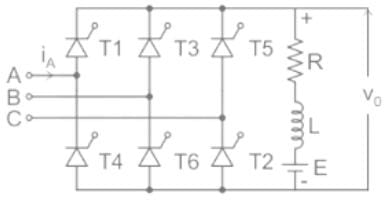• A three-phase input supply is connected to the terminal A, B, C, and RLE load is connected across the output terminal.
• It worked as three-phase AC to DC converter for firing angle delay 0 <α ≤ 90.
• The positive group of SCRs is fired at an interval of 120° and a similarly negative group of SCRs fired at an interval of 120°, but SCR of both groups are fired at an internal of 60° or commutation occurs at every 60°.
• At any time tow SCRs, one from the positive group and one from the negative group must be conducted together for the source to energize the load.

Waveform: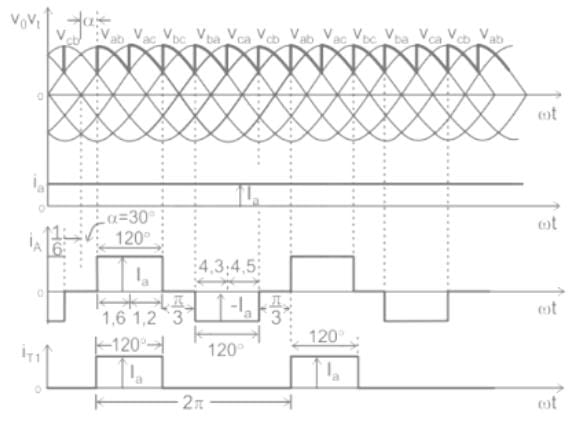Voltage and Current Equation:

The average output voltage (V0) is given by,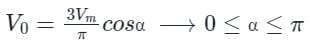Where Vm is the maximum value of line voltage.

Voltage and current waveform for α = 30° and for constant current can be drawn as,

From waveform RMS value of Thyristor current (IT = iT1) will be,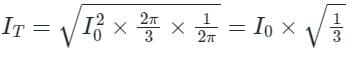Where I0 is constant DC load current.

Test: Phase Controlled Rectifiers - Question 2

### In a single Phase full converter with resistive load and firing angle α. The load current is non-zero and zero, respectively for-

Detailed Solution for Test: Phase Controlled Rectifiers - Question 2

Concept:

Phase-controlled converters:

• It is a device which is used to convert A.C sinusoidal into D.C.
• In this the A.C input is fed from transformer stepdown side and then input is converted into D.C output.
• Here we are talking about single phase fully controlled converter.

Single phase full converter with resistive load: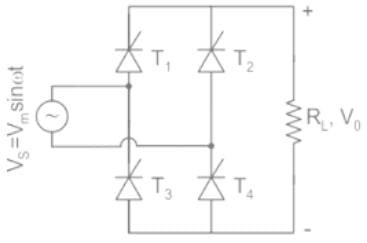• An A.C voltage source of Vs = Vmsin(wt) is connected.
• Four controlled thyristors and a resistive load is connected.
• Let Vo be the output voltage.

Working:

• Let thyristor T1 and T4 are triggered at a firing angle of 'α'.
• During the first cycle from '0 to π' the current starts flowing at firing 'αand flows through Vs→ T1​→ RL​→ T4​→ Vs.
• During the second cycle from 'π→2π' the thyristor T2 and T3 are triggered at (π + α)
• The current flows through Vs→ T​2→ RL​→ T3​→ Vs.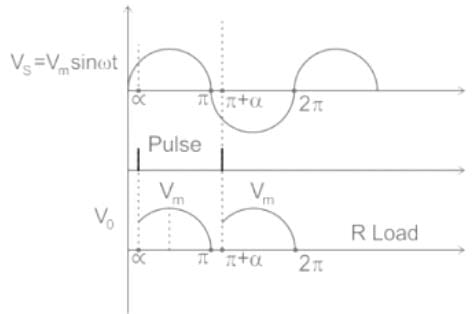Current flowing through a resistive load will be

I = Vo/R

We can see from the above graph that the current will be zero from '0 to α' and the current will be non-zero from α to π.

So, the current will be non-zero and zero from 'π - α' and 'α' respectively.

*Answer can only contain numeric values
Test: Phase Controlled Rectifiers - Question 3

### 3 phase, 6 pulse converter shown in the figure, the load is taking ripple free constant current of 10 A. The average output voltage of the converter is 150 V at a firing angle α = 30º. Find the value of line resistance R in ohms. (Up to two decimal places)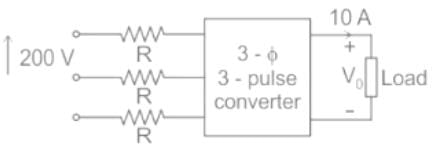Detailed Solution for Test: Phase Controlled Rectifiers - Question 3

Concept:

The average output voltage of 3 phase 6 pulse converter is given by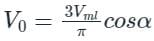here Vml is the maximum value of line-to-line voltage

The voltage drop in the resistance is given as

2RI0, here 2 resistance will come in to picture in every 60º conduction of two phases

so this voltage drop is subtracted from the final output of this converter.

Solution:

The average output voltage is equal to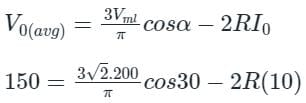R = 4.19 Ω

Test: Phase Controlled Rectifiers - Question 4

In a dual converter, if the firing angle of one bridge is 30°, then the firing angle of second bridge is ______.

Detailed Solution for Test: Phase Controlled Rectifiers - Question 4

Concept:

• A dual converter is an electronic converter or circuit which comprises of two converters.
• One performs as a rectifier and the other performs as the inverter.
• Two full converters are arranged in an anti-parallel pattern and linked to the same DC load.
• These converters can provide four-quadrant operations.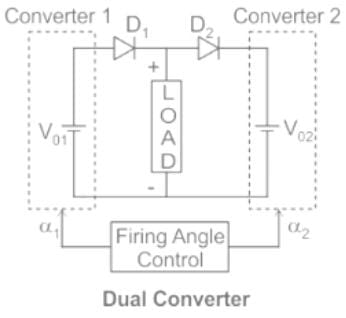Modes of operation of Dual converter:

Non-circulating current mode:

• There is no circulating current between the converters in this mode as only one converter will perform at a time
• During converter 1 operation, firing angle (α1) will be between 0 to 90°
• During converter 2 operation, firing angle (α2) will be between 0 and 90°

Circulating current Mode:

• In this mode, there is a circulating current as the two converters will be in the ON condition at the same time.
• The firing angles are adjusted such that
• Firing angle of converter 1 (α1) + firing angle of converter 2 (α2) = 180°
• Converter 1 as rectifier when 0° < α1 < 90° and converter 2 as inverter when 90° < α2 < 180°
• Converter 1 as inverter when 90° < α1 < 180° and converter 2 as rectifier when 0° < α2 < 90°
• Four quadrant operation of dual converter is shown below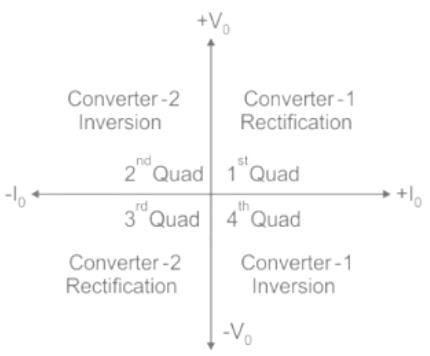Application of dual converter:

• Direction and speed control of DC motors
• Applicable wherever the reversible DC required
• Industrial variable speed DC drives.

Calculation:

Given that, firing angle of one bridge is 30° (i.e. α1 = 30°)

We know that firing angles can never be greater than 180°.

i.e. α1 + α2 = 180°

∴ 30° + α2 = 180°

α2 = 180° - 30°

α2 = 150°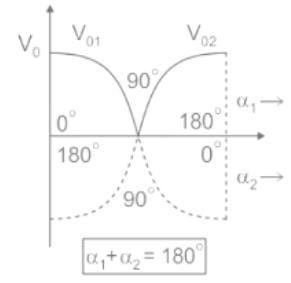Test: Phase Controlled Rectifiers - Question 5

A delayed full-wave rectified sinusoidal current has an average value equal to half its maximum value. Find the delay angle θ.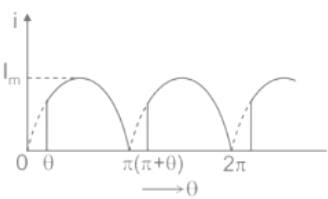Detailed Solution for Test: Phase Controlled Rectifiers - Question 5

Concept:

The average output voltage of a full-wave controlled rectifier with R load is given by: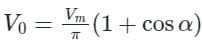Where Vm is the maximum value of supply voltage

α is the firing angle or delay angle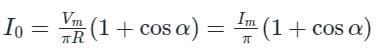Calculation:

Given that, the average value of the average load current is equal to half of its maximum value.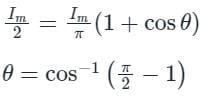Test: Phase Controlled Rectifiers - Question 6

A delayed full-wave rectified sinusoidal current has an average value equal to one – third its maximum value. Find the delay angle.

Detailed Solution for Test: Phase Controlled Rectifiers - Question 6

Concept:

Considered Vm is the maximum value of AC input voltage of converter and V0 is the average output voltage converter and α is delay angle.

For single-phase semi converter or delayed full-wave rectifier,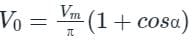Calculation:

Given that,

V0 = Vm/3

Hence, the equation becomes,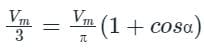or,
π/3 = (1 + cosα)
cos α = 0.047
α = cos-1(0.047)

Test: Phase Controlled Rectifiers - Question 7

Distortion factor (DF) and total harmonic distortion (THD) are related by.

Detailed Solution for Test: Phase Controlled Rectifiers - Question 7

Total harmonic distortion:

• It is a common measurement of the level of harmonic distortion present in power systems.
• THD can be related to either current harmonics or voltage harmonics.
• It is defined as the ratio of r.m.s value of all the harmonic components to the r.m.s value of the fundamental component.
• Mathematically, it can be represented as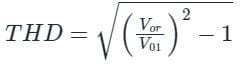Where,
Vor = Total rms value

01 = Fundamental rms value

Distortion factor (DF): It is the ratio of the fundamental component to r.m.s value of the waveform.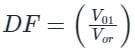Distortion factor (DF) and total harmonic distortion (THD) are related as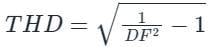Test: Phase Controlled Rectifiers - Question 8

A freewheeling diode in a phase-controlled rectifier:

Detailed Solution for Test: Phase Controlled Rectifiers - Question 8

Freewheeling diode:

• A freewheeling diode placed across the inductive load will provide a path for the release of energy stored in the inductor while the load voltage drops to zero.
• The freewheeling diode prevents the load voltage from becoming negative. Whenever load voltage tends to go negative, FD comes into play.
• As a result, the load current is transferred from the main thyristor to FD, allowing the thyristor to regain its forward blocking capability.
• The advantages are the input power factor is improved, load current waveform is improved and better load performance.
Test: Phase Controlled Rectifiers - Question 9

A single-phase, full-bridge diode rectifier fed from a 230 V, 50 Hz sinusoidal source supplies a series combination of finite resistance, R, and a very large inductance, L. The two most dominant frequency components in the source current are:

Detailed Solution for Test: Phase Controlled Rectifiers - Question 9

Concept:

Fourier series of source current in a full bridge rectifier is given by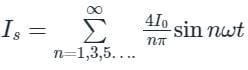For n = 1, 3, 5, ......

The most dominant frequency components in the above expression are f, 3f

Application:

A single-phase, full-bridge diode rectifier fed from a 230 V, 50 Hz sinusoidal source

Dominant frequencies = f, 3f

= 50 and 150 Hz

Test: Phase Controlled Rectifiers - Question 10

A six-pulse thyristor bridge rectifier is connected to a balanced three-phase, 50 Hz AC source. Assuming that the DC output current of the rectifier is constant, the lowest harmonic component in the AC input current is

Detailed Solution for Test: Phase Controlled Rectifiers - Question 10

In a six-pulse thyristor bridge rectifier, the harmonics present are = 6 k ± 1

So, the harmonics are = 5, 7, 11, 13, ...

lowest harmonic component = 5th harmonic supply frequency = 50 Hz

5th harmonic frequency = 5f = 250 Hz

## Power Electronics

5 videos|39 docs|63 tests
 Use Code STAYHOME200 and get INR 200 additional OFF Use Coupon Code
Information about Test: Phase Controlled Rectifiers Page
In this test you can find the Exam questions for Test: Phase Controlled Rectifiers solved & explained in the simplest way possible. Besides giving Questions and answers for Test: Phase Controlled Rectifiers, EduRev gives you an ample number of Online tests for practice

## Power Electronics

5 videos|39 docs|63 tests